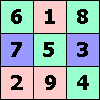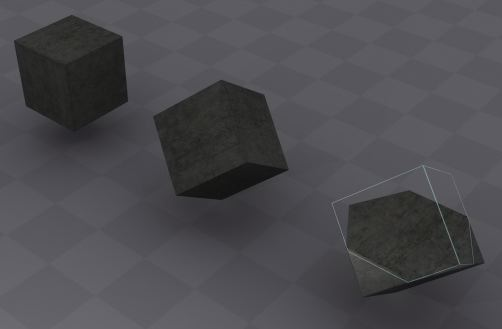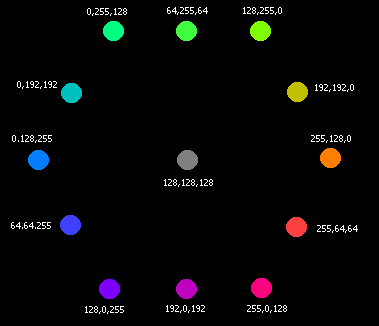ONE TO NINE by Andrew HodgesPage 71: This page explains the confusion surrounding the word 'billion' from its earliest days. This BBC report has an example of the frequent confusion of billions with mere millions. The Iraq billions, just a drop in the ocean...

A website on Paper Money Trivia brings out that the largest denomination ever issued as a banknote was for 1020 Pengo, in Hungary, 1946. It was worth a few pennies.

Incidentally the word 'trivia' comes from the THREE medieval subjects of grammar, logic and rhetoric.

As a further trivial comment, Tony Judt's history Postwar: A history of Europe since 1945 describes the Hungarian inflation of 1946 as peaking with the pengo at "5 quintillion (530) to the dollar". Large numbers and powers are definitely rather confusing...

Page 72:

210 ≈ 1000, so
220 ≈ 1000000, a million, 106;
230 ≈ 1000000000, a billion, 109;
240 ≈ 1000000000000, a trillion, 1012
and then 280 ≈ a trillion trillion, 1024.

Page 75:

81 is 34, so 80 ≈ 81 implies that
312 ≈ 803
Now use the approximation 312 ≈ 219 to give
219 ≈ 803 = 53 × 212.
Dividing by 29,
210 ≈ 53 × 23 = 103, i.e. 1024 ≈ 1000.

A perfect harmonic seventh is given by the ratio 7/4. A perfect harmonic fourth is given by the ratio 4/3. Two fourths give 16/9, and the approximation 16/9 ≈ 7/4 is equivalent to 64 ≈ 63.Page 79:

Hexadecimal FF means 255 in decimal notation, as already explained. Then 69 in hexadecimal means six sixteens plus nine, which is 105 in decimal notation. Hexadecimal B4 means eleven sixteens plus four, which is 180.

The number of different colours is 256 × 256 × 256 = (28)3 = 224 ≈ 16 million.Page 80:

It may come as a surprising fact that when a cube is suspended from one vertex, and then bisected by a horizontal plane, the cut makes a regular hexagon.

This graphic showing the hexagon was created by John Augar.This shows the plane of colours with R + G + B = 384, forming the central hexagon.
Page 83:

Three dimensions for the location of the camera. Two for the direction in which the lens points, one more for the angle of the shot, totalling six. If you limit yourself to pictures which respect the vertical direction, however, only five. For the spotlight, another three dimensions for location and two for the direction of the shot. If it has a shade with some asymmetry, then there is another dimension.

Page 89: The Millennium Prize problems.